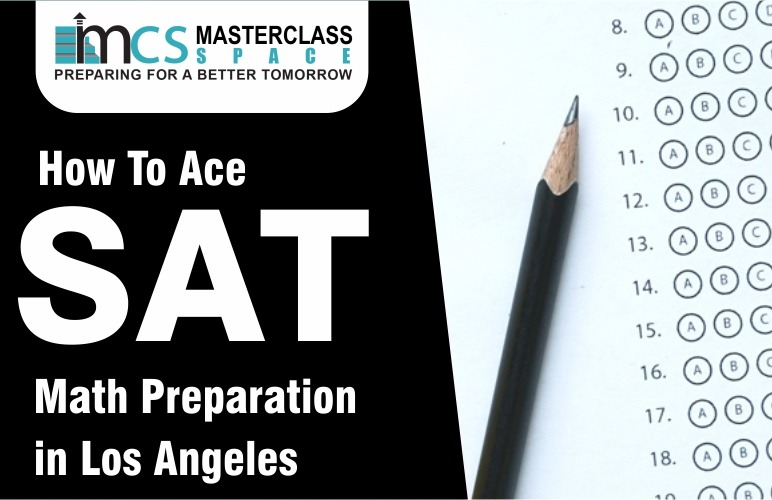# How to ace SAT Math Preparation in Los Angeles?There is no better SAT preparation in Los Angeles than Masterclass Space. All of our world-class tutors, who have a proven track record of success, teach & work tirelessly to maintain Masterclass Space’s reputation for offering the Best Sat Classes in Los Angeles. Using a simple formula of one-on-one training, easily accessible & interactive online courses, and SAT practice exams, we achieve this goal.

Areas on the SAT Math Section that are tested

The SAT Math section is divided into three sections: Algebra, Advanced Math, and Problem Solving and Data Analysis. You will not find calculus-level math on the SAT Math exam. But, Geometry and Algebra II are the primary sources of difficulties. Theorems of geometry, types of graphs, and what each point on the graph means are all things that you should know before tackling the problems. These concepts make up about 80% of the SAT questions. Then there’s the option of brushing up on concepts like circles and radians as well as probabilities such as rates and ratios. These ideas form the basis of the remaining 80%. The math principles themselves are not tough, but it can be difficult to know which concepts to utilize to answer a particular topic. There will be a lot of word problems evaluating your ability to reason logically. Those who have completed calculus but are unable to choose which technique to employ in specific questions on the SAT will not receive a full score. Improve your arithmetic skills, including mental math (for rapid estimation), fractions, and decimal points. You’ll be able to focus on the more difficult questions if you don’t have to worry about them. All in all, you will need to concentrate on the fundamentals of algebra, as well as the subjects discussed above.7 Tips for Best SAT Preparation in Los Angeles

More Practice

The more time you spend doing arithmetic problems, the better you get. It’s critical to solve the concept problems multiple times before taking the exam. There is no getting around the fact that to do well in SAT math section, you must have done a lot of math questions beforehand.

Review Questions

There may be exceedingly complex problems, requiring numerous stages to solve.

If you don’t write down all the steps, you can get the wrong answer. This is vital in the non-calculator portion where you can’t use a calculator to determine the answer. Write down your steps so you don’t miss a step and miss a question!

Learn the Basics

Please keep in mind that mathematics is a sequential subject and that it is essential to have a thorough grasp of the fundamental concepts that drive a mathematical issue before proceeding to work on more difficult solutions. Focus on understanding the process and logic involved instead of memorizing the process.

Memorizing Important Formulas

You’d be stumped if you didn’t know your trigonometry formulas. It’s impossible to answer without knowing the sine and cosine of an angle. Memorizing formulas is not only important for some issues, but it can also save time.

Combine two or more ideas to solve a problem

The no-calculator parts of the new SAT were added to make sure that students can do simple arithmetic, which college professors say is important in most academic areas and is also important in many professional and everyday situations. It tests two main areas: Heart of Algebra and Passport to Advanced Math, along with problems that fall under Additional Topics. There is no calculator in this section which will require combining ideas to solve a problem. These categories are about things like linear equations, linear inequalities, functions, quadratic equations, graphs, geometry, and complex numbers.

Avoid Careless Mistakes